Last week Warren Kuhfeld wrote about a graph called the "lines plot" that is produced by SAS/STAT procedures in SAS 9.4M5. (Notice that the "lines plot" has an 's'; it is not a line plot!) The lines plot is produced as part of an analysis that performs multiple comparisons of means. Although the graphical version of the lines plot is new in SAS 9.4M5, the underlying analysis has been available in SAS for decades. If you have an earlier version of SAS, the analysis is presented as a table rather than as a graph.

Warren's focus was on the plot itself, with an emphasis on how to create it. However, the plot is also interesting for the statistical information it provides. This article discusses how to interpret the lines plot in a multiple comparisons of means analysis.

### The lines plot in SAS

You can use the LINES option in the LSMEANS statement to request a lines plot in SAS 9.4M5. The following data are taken from Multiple Comparisons and Multiple Tests (p. 42-53 of the First Edition). Researchers are studying the effectiveness of five weight-loss diets, denoted by A, B, C, D, and E. Ten male subjects are randomly assigned to each method. After a fixed length of time, the weight loss of each subject is recorded, as follows:

```/* Data and programs from _Multiple Comparisons and Multiple Tests_ Westfall, Tobias, Rom, Wolfinger, and Hochberg (1999, First Edition) */ data wloss; do diet = 'A','B','C','D','E'; do i = 1 to 10; input WeightLoss @@; output; end; end; datalines; datalines; 12.4 10.7 11.9 11.0 12.4 12.3 13.0 12.5 11.2 13.1 9.1 11.5 11.3 9.7 13.2 10.7 10.6 11.3 11.1 11.7 8.5 11.6 10.2 10.9 9.0 9.6 9.9 11.3 10.5 11.2 8.7 9.3 8.2 8.3 9.0 9.4 9.2 12.2 8.5 9.9 12.7 13.2 11.8 11.9 12.2 11.2 13.7 11.8 11.5 11.7 ;```

You can use PROC GLM to perform a balanced one-way analysis of variance and use the MEANS or LSMEANS statement to request pairwise comparisons of means among the five diet groups:

```proc glm data=wloss; class diet; model WeightLoss = diet; *means diet / tukey LINES; lsmeans diet / pdiff=all adjust=tukey LINES; quit;```

In general, I use the LSMEANS statement rather than the MEANS statement because LS-means are more versatile and handle unbalanced data. (More about this in a later section.) The PDIFF=ALL option requests an analysis of all pairwise comparisons between the LS-means of weight loss for the different diets. The ADJUST=TUKEY option is a common way to adjust the widths of confidence intervals to accommodate the multiple comparisons. The analysis produces several graphs and tables, including the following lines plot.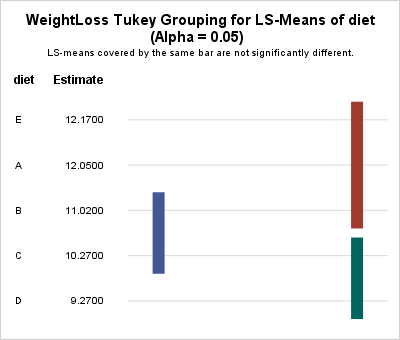### How to interpret a lines plot

In the lines plot, the vertical lines visually connect groups whose LS-means are "statistically indistinguishable." Statistically speaking, two means are "statistically indistinguishable" when their pairwise difference is not statistically significant.

If you have k groups, there are k(k-1)/2 pairwise differences that you can examine. The lines plot attempts to summarize those comparisons by connecting groups whose means are statistically indistinguishable. Often there are fewer lines than pairwise comparisons, so the lines plot displays a summary of which groups have similar means.

In the previous analysis, there are five groups, so there are 10 pairwise comparisons of means. The lines plot summarizes the results by using three vertical lines. The leftmost line (blue) indicates that the means of the 'B' and 'C' groups are statistically indistinguishable (they are not significantly different). Similarly, the upper right vertical bar (red) indicates that the means of the pairs ('E','A'), ('E','B'), and ('A','B') are not significantly different from each other. Lastly, the lower right vertical bar (green) indicates that the means for groups 'C' and 'D' are not significantly different. Thus in total, the lines plot indicates that five pairs of means are not significantly different.

The remaining pairs of mean differences (for example, 'E' and 'D') are significantly different. By using only three vertical lines, the lines plot visually associates pairs of means that are essentially the same. Those pairs that are not connected by a vertical line are significantly different.

### Advantages and limitations of the lines plot

Advantages of the lines plot include the following:

• The groups are ordered according to the observed means of the groups.
• The number of vertical lines is often much smaller than the number of pairwise comparisons between groups.

Notice that the groups in this example are the same size (10 subjects). When the group sizes are equal (the so-called "balanced ANOVA" case), the lines plot can always correctly represent the relationships between the group means. However, that is not always true for unbalanced data. Westfall et al. (1999, p. 69) provide an example in which using the LINES option on the MEANS statement produces a misleading plot.

The situation is less severe when you use the LSMEANS statement, but for unbalanced data it is sometimes impossible for the lines plot to accurately connect all groups that have insignificant mean differences. In those cases, SAS appends a footnote to the plot that alerts you to the situation and lists the additional significances not represented by the plot.

In my next blog post, I will show some alternative graphical displays that are appropriate for multiple comparisons of means for unbalanced groups.

### Summary

In summary, the new lines plot in SAS/STAT software is a graphical version of an analysis that has been in SAS for decades. You can create the plot by using the LINES option in the LSMEANS statement. The lines plot indicates which groups have mean differences that are not significant. For balanced data (or nearly balanced), it does a good job of summarizes which differences of means are not significant. For highly unbalanced data, there are other graphs that you can use. Those graphs will be discussed in a future article.

The post Graphs for multiple comparisons of means: The lines plot appeared first on The DO Loop.Correlations between variables are typically displayed in a matrix. Because the correlation matrix is determined by the order of the variables, it is difficult to find the largest and smallest correlations, which is why analysts sometimes use colors to visualize the correlation matrix. Another visualization option is the pairwise correlation plot, which orders pairs of variables by their correlations.

Neither graph addresses a related problem: for each variable, which other variables are strongly correlated with it? Which are weakly correlated? In SAS, the CORR procedure supports a little-known option that answers that question. You can use the RANK option in the PROC CORR statement to order the correlations for each variable (independently) according to the magnitude of the correlations.

Notice that the RANK option does not compute "rank correlation." You can compute rank correlation by using the SPEARMAN option.

### Ordering correlations by size

Consider the Sashelp.Heart data set, which contains data for 5209 patients who enrolled in the Framingham Heart Study. You might want to know which variables are highly correlated with weight, or smoking, or blood pressure, to name a few examples. The following call to PROC CORR uses the RANK option to order each row of correlations in the output:

```/* Note: The MRW variable is similar to the body-mass index (BMI). */ proc corr data=sashelp.Heart RANK noprob; var Height Weight MRW Smoking Diastolic Systolic Cholesterol; run;```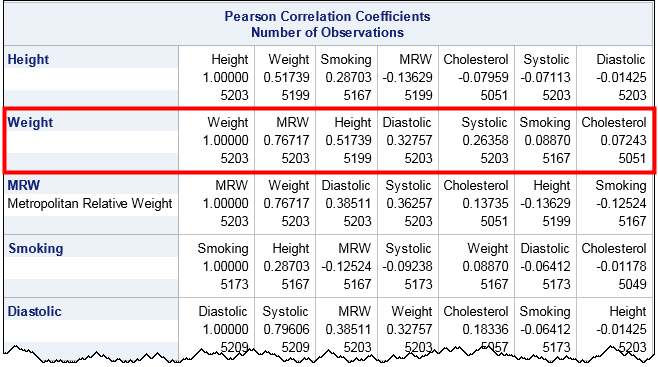The output orders each row according to the magnitude of the correlations. (Click to enlarge.) For example, look at the row for the Weight variable, which is highlighted by a red rectangle. Scanning across the row, you can see that the variables that are the most strongly correlated with Weight are MRW (which measures whether a patient is overweight) and the height. At the end of the row are the variables that are essentially uncorrelated with Weight, namely Smoking and Cholesterol. The numbers at the bottom of each cell indicate the number of nonmissing pairwise observations.

In a similar way, look at the row for the Smoking variable. That variable is most strongly correlated with Height and MRW. Notice that the correlation with MRW is negative, which shows that the correlations are ordered by absolute values (magnitude). The highly correlated variables—whether positively or negatively correlated—appear first and the uncorrelated variable (correlations near zero) occur last in each row.

### Ordering correlations for groups of variables

It is common to want to examine the correlations between groups of variables. For example, a clinician might want to look at the correlations between clinical measurements (blood pressure, cholesterol,...) and genetic or lifestyle choices (weight, smoking habits,...). The following call to PROC CORR uses the VAR and WITH statements to compare groups of variables, and uses the RANK option to order the correlations along each row:

```proc corr data=sashelp.Heart RANK noprob; var Height Weight MRW Smoking; /* genetic and lifestyle factors */ with Diastolic Systolic Cholesterol; /* clinical measurements */ run;```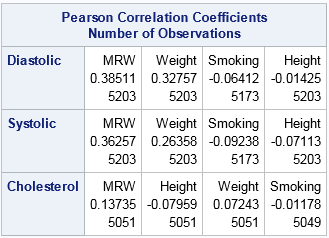Notice that the number of variables in the VAR statement determine the columns. The variables in the WITH statement determine the rows. Within each row, the variables in the VAR statement are ordered by the magnitude of the correlation. For these data, the Diastolic and Systolic variables are similar with respect to how they correlate with the column variables. The order of the column variables is the same for the first two rows. In contrast, the strength of the correlations between the Cholesterol variable and the column variables are in a different order.

In summary, you can use the RANK option in the PROC CORR statement to order the rows of a correlation matrix according to the magnitude (absolute value) of the correlations between each variable and the others. This makes it easy to find pairs of variables that are strongly correlated and pairs that are weakly correlated.

The post Order correlations by magnitude appeared first on The DO Loop.If you perform a weighted statistical analysis, it can be useful to produce a statistical graph that also incorporates the weights. This article shows how to construct and interpret a weighted histogram in SAS.

### How to construct a weighted histogram

Before constructing a weighted histogram, let's review the construction of an unweighted histogram. A histogram requires that you specify a set of evenly spaced bins that cover the range of the data. An unweighted histogram of frequencies is constructed by counting the number of observations that are in each bin. Because counts are dependent on the sample size, n, histograms often display the proportion (or percentage) of values in each bin. The proportions are the counts divided by n. On the proportion scale, the height of each bin is the sum of the quantity 1/n, where the sum is taken over all observations in the bin.

That fact is important because it reveals that the unweighted histogram is a special case of the weighted histogram. An unweighted histogram is equivalent to a weighted histogram in which each observation receives a unit weight. Therefore the quantity 1/n is the standardized weight of each observation: the weight divided by the sum of the weights. The formula is the same for non-unit weights: the height of each bin is the sum of the quantity wi / Σ wi, where the sum is taken over all observations in the bin. That is, you add up all the standardized weights in each bin to produce the bin height.

### An example of a weighted histogram

The SAS documentation for the WEIGHT statement includes the following example. Twenty subjects estimate the diameter of an object that is 30 cm across. Some people are placed closer to the object than others. The researcher believes that the precision of the estimate is inversely proportional to the distance from the object. Therefore the researcher weights each subject's estimate by using the inverse distance.

The following DATA step creates the data, and PROC SGPLOT creates a weighted histogram of the data by using the WEIGHT= option on the HISTOGRAM option. (The WEIGHT= option was added in SAS 9.4M1.)

```data Size; input Distance ObjectSize @@; Wt = 1 / distance; /* precision */ x = ObjectSize; label x = "Estimate of Size"; datalines; 1.5 30 1.5 20 1.5 30 1.5 25 3 43 3 33 3 25 3 30 4.5 25 4.5 36 4.5 48 4.5 33 6 43 6 36 6 23 6 48 7.5 30 7.5 25 7.5 50 7.5 38 ;   title "Weighted Histogram of Size Estimate"; proc sgplot data=size noautolegend; histogram x / WEIGHT=Wt scale=proportion datalabel binwidth=5; fringe x / lineattrs=(thickness=2 color=black) transparency=0.6; yaxis grid offsetmin=0.05 label="Weighted Proportion"; refline 30 / axis=x lineattrs=(pattern=dash); run;```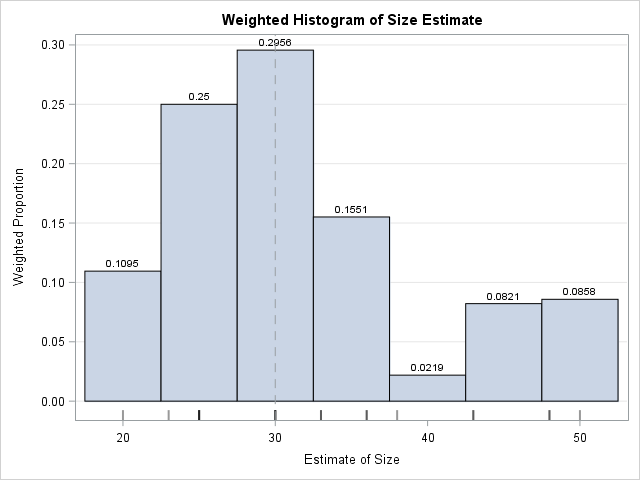The weighted histogram is shown to the right. The data values are shown in the fringe plot beneath the histogram. The height of each bin is the sum of the weights of the observations in that bin. The dashed line represents the true diameter of the object. Most estimates are clustered around the true value, except for a small cluster of larger estimates. Notice that I use the SCALE=PROPORTION option to plot the weighted proportion of observations in each bin, although the default behavior (SCALE=PERCENT) would also be acceptable.

If you remove the WEIGHT= option and study the unweighted graph, you will see that the average estimate for the unweighted distribution (33.6) is not as close to the true diameter as the weighted estimate (30.1). Furthermore, the weighted standard deviation is about half the unweighted standard deviation, which shows that the weighted distribution of these data has less variance than the unweighted distribution.

By the way, although PROC UNIVARIATE can produce weighted statistics, it does not create weighted graphics as of SAS 9.4M5. One reason is that the graphics statements (CDFPLOT, HISTOGRAM, QQPLOT, etc) not only create graphs but also fit distributions and produce goodness-of-fit statistics, and those analyses do not support weight variables.

### Checking the computation

Although a weighted histogram is not conceptually complex, I understand a computation better when I program it myself. You can write a SAS program that computes a weighted histogram by using the following algorithm:

1. Construct the bins. For this example, there are eight bins of width 5, and the first bin starts at x=17.5. (It is centered at x=20.) Initialize all bin heights to zero.
2. For each observation, find the bin that contains it. Increment the bin height by the weight of that observation.
3. Standardize the heights by dividing by the sum of weights. You can skip this step if the weights sum to unity.

A SAS/IML implementation of this algorithm requires only a few lines of code. A DATA step implementation that uses arrays is longer, but probably looks more familiar to many SAS programmers:

```data BinHeights(keep=height:); array EndPt _temporary_; binStart = 17.5; binWidth = 5; /* anchor and width for bins */ do i = 1 to dim(EndPt); /* define endpoints of bins */ EndPt[i] = binStart + (i-1)*binWidth; end;   array height; /* height of each bin */ set Size end=eof; /* for each observation ... */ sumWt + Wt; /* compute sum of weights */ Found=0; do i = 1 to dim(EndPt)-1 while (^Found); /* find bin for each obs */ Found = (EndPt[i] <= x < EndPt[i+1]); if Found then height[i] + Wt; /* increment bin height by weight */ end; if eof then do; do i = 1 to dim(height); /* scale heights by sum of weights */ height[i] = height[i] / sumWt; end; output; end; run;   proc print noobs data=BinHeights; run;```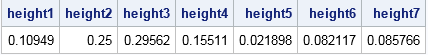The computations from the DATA step match the data labels that appear on the weighted histogram in PROC SGPLOT.

### Summary

In SAS, the HISTOGRAM statement in PROC SGPLOT supports the WEIGHT= option, which enables you to create a weighted histogram. A weighted histogram shows the weighted distribution of the data. If the histogram displays proportions (rather than raw counts), then the heights of the bars are the sum of the standardized weights of the observations within each bin. You can download the SAS program that computes the quantities in this article.

How can you interpret a weighted histogram? That depends on the meaning of the weight variables. For survey data and sampling weights, the weighted histogram estimates the distribution of a quantity in the population. For inverse variance weights (such as were used in this article), the weighted histogram overweights precise measurements and underweights imprecise measurements. When the weights are correct, the weighted histogram is a better estimate of the density of the underlying population and the weighted statistics (mean, variance, quantiles,...) are better estimates of the corresponding population quantities.

Have you ever plotted a weighted histogram? What was the context? Leave a comment.

The post Create and interpret a weighted histogram appeared first on The DO Loop.If you perform a weighted statistical analysis, it can be useful to produce a statistical graph that also incorporates the weights. This article shows how to construct and interpret a weighted histogram in SAS.

### How to construct a weighted histogram

Before constructing a weighted histogram, let's review the construction of an unweighted histogram. A histogram requires that you specify a set of evenly spaced bins that cover the range of the data. An unweighted histogram of frequencies is constructed by counting the number of observations that are in each bin. Because counts are dependent on the sample size, n, histograms often display the proportion (or percentage) of values in each bin. The proportions are the counts divided by n. On the proportion scale, the height of each bin is the sum of the quantity 1/n, where the sum is taken over all observations in the bin.

That fact is important because it reveals that the unweighted histogram is a special case of the weighted histogram. An unweighted histogram is equivalent to a weighted histogram in which each observation receives a unit weight. Therefore the quantity 1/n is the standardized weight of each observation: the weight divided by the sum of the weights. The formula is the same for non-unit weights: the height of each bin is the sum of the quantity wi / Σ wi, where the sum is taken over all observations in the bin. That is, you add up all the standardized weights in each bin to produce the bin height.

### An example of a weighted histogram

The SAS documentation for the WEIGHT statement includes the following example. Twenty subjects estimate the diameter of an object that is 30 cm across. Some people are placed closer to the object than others. The researcher believes that the precision of the estimate is inversely proportional to the distance from the object. Therefore the researcher weights each subject's estimate by using the inverse distance.

The following DATA step creates the data, and PROC SGPLOT creates a weighted histogram of the data by using the WEIGHT= option on the HISTOGRAM option. (The WEIGHT= option was added in SAS 9.4M1.)

```data Size; input Distance ObjectSize @@; Wt = 1 / distance; /* precision */ x = ObjectSize; label x = "Estimate of Size"; datalines; 1.5 30 1.5 20 1.5 30 1.5 25 3 43 3 33 3 25 3 30 4.5 25 4.5 36 4.5 48 4.5 33 6 43 6 36 6 23 6 48 7.5 30 7.5 25 7.5 50 7.5 38 ;   title "Weighted Histogram of Size Estimate"; proc sgplot data=size noautolegend; histogram x / WEIGHT=Wt scale=proportion datalabel binwidth=5; fringe x / lineattrs=(thickness=2 color=black) transparency=0.6; yaxis grid offsetmin=0.05 label="Weighted Proportion"; refline 30 / axis=x lineattrs=(pattern=dash); run;```The weighted histogram is shown to the right. The data values are shown in the fringe plot beneath the histogram. The height of each bin is the sum of the weights of the observations in that bin. The dashed line represents the true diameter of the object. Most estimates are clustered around the true value, except for a small cluster of larger estimates. Notice that I use the SCALE=PROPORTION option to plot the weighted proportion of observations in each bin, although the default behavior (SCALE=PERCENT) would also be acceptable.

If you remove the WEIGHT= option and study the unweighted graph, you will see that the average estimate for the unweighted distribution (33.6) is not as close to the true diameter as the weighted estimate (30.1). Furthermore, the weighted standard deviation is about half the unweighted standard deviation, which shows that the weighted distribution of these data has less variance than the unweighted distribution.

By the way, although PROC UNIVARIATE can produce weighted statistics, it does not create weighted graphics as of SAS 9.4M5. One reason is that the graphics statements (CDFPLOT, HISTOGRAM, QQPLOT, etc) not only create graphs but also fit distributions and produce goodness-of-fit statistics, and those analyses do not support weight variables.

### Checking the computation

Although a weighted histogram is not conceptually complex, I understand a computation better when I program it myself. You can write a SAS program that computes a weighted histogram by using the following algorithm:

1. Construct the bins. For this example, there are eight bins of width 5, and the first bin starts at x=17.5. (It is centered at x=20.) Initialize all bin heights to zero.
2. For each observation, find the bin that contains it. Increment the bin height by the weight of that observation.
3. Standardize the heights by dividing by the sum of weights. You can skip this step if the weights sum to unity.

A SAS/IML implementation of this algorithm requires only a few lines of code. A DATA step implementation that uses arrays is longer, but probably looks more familiar to many SAS programmers:

```data BinHeights(keep=height:); array EndPt _temporary_; binStart = 17.5; binWidth = 5; /* anchor and width for bins */ do i = 1 to dim(EndPt); /* define endpoints of bins */ EndPt[i] = binStart + (i-1)*binWidth; end;   array height; /* height of each bin */ set Size end=eof; /* for each observation ... */ sumWt + Wt; /* compute sum of weights */ Found=0; do i = 1 to dim(EndPt)-1 while (^Found); /* find bin for each obs */ Found = (EndPt[i] <= x < EndPt[i+1]); if Found then height[i] + Wt; /* increment bin height by weight */ end; if eof then do; do i = 1 to dim(height); /* scale heights by sum of weights */ height[i] = height[i] / sumWt; end; output; end; run;   proc print noobs data=BinHeights; run;```The computations from the DATA step match the data labels that appear on the weighted histogram in PROC SGPLOT.

### Summary

In SAS, the HISTOGRAM statement in PROC SGPLOT supports the WEIGHT= option, which enables you to create a weighted histogram. A weighted histogram shows the weighted distribution of the data. If the histogram displays proportions (rather than raw counts), then the heights of the bars are the sum of the standardized weights of the observations within each bin. You can download the SAS program that computes the quantities in this article.

How can you interpret a weighted histogram? That depends on the meaning of the weight variables. For survey data and sampling weights, the weighted histogram estimates the distribution of a quantity in the population. For inverse variance weights (such as were used in this article), the weighted histogram overweights precise measurements and underweights imprecise measurements. When the weights are correct, the weighted histogram is a better estimate of the density of the underlying population and the weighted statistics (mean, variance, quantiles,...) are better estimates of the corresponding population quantities.

Have you ever plotted a weighted histogram? What was the context? Leave a comment.

The post Create and interpret a weighted histogram appeared first on The DO Loop.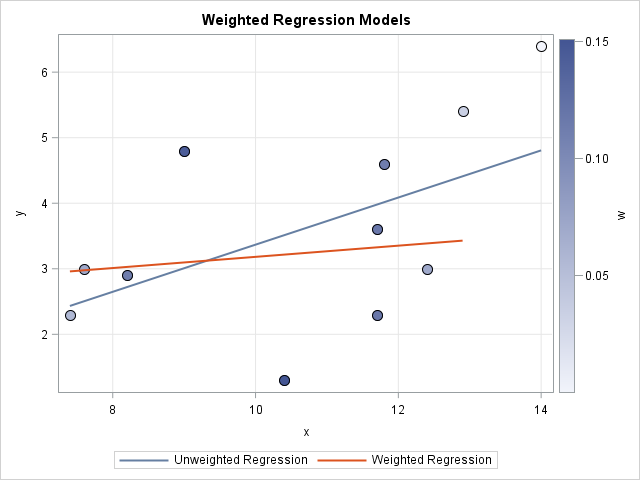How can you specify weights for a statistical analysis? Hmmm, that's a "weighty" question! Many people on discussion forums ask "What is a weight variable?" and "How do you choose a weight for each observation?" This article gives a brief overview of weight variables in statistics and includes examples of how weights are used in SAS.

### Different kinds of weight variables

One source of confusion is that different areas of statistics use weights in different ways. All weights are not created equal! The weights in survey statistics have a different interpretation from the weights in a weighted least squares regression.

Let's start with a basic definition. A weight variable provides a value (the weight) for each observation in a data set. The i_th weight value, wi, is the weight for the i_th observation. For most applications, a valid weight is nonnegative. A zero weight usually means that you want to exclude the observation from the analysis. Observations that have relatively large weights have more influence in the analysis than observations that have smaller weights. An unweighted analysis is the same as a weighted analysis in which all weights are 1.

There are several kinds of weight variables in statistics. At the 2007 Joint Statistical Meetings in Denver, I discussed weighted statistical graphics for two kinds of statistical weights: survey weights and regression weights. An audience member informed me that STATA software provides four definitions of weight variables, as follows:

• Frequency weights: A frequency variable specifies that each observation is repeated multiple times. Each frequency value is a nonnegative integer.
• Survey weights: Survey weights (also called sampling weights or probability weights) indicate that an observation in a survey represents a certain number of people in a finite population. Survey weights are often the reciprocals of the selection probabilities for the survey design.
• Analytical weights: An analytical weight (sometimes called an inverse variance weight or a regression weight) specifies that the i_th observation comes from a sub-population with variance σ2/wi, where σ2 is a common variance and wi is the weight of the i_th observation. These weights are used in multivariate statistics and in a meta-analyses where each "observation" is actually the mean of a sample.
• Importance weights: According to a STATA developer, an "importance weight" is a STATA-specific term that is intended "for programmers, not data analysts." The developer says that the formulas "may have no statistical validity" but can be useful as a programming convenience. Although I have never used STATA, I imagine that a primary use is to downweight the influence of outliers. an example that shows the distinction between a frequency variable and a weight variable in regression. Briefly, a frequency variable is a notational convenience that enables you to compactly represent the data. A frequency variable determines the sample size (and the degrees of freedom), but using a frequency variable is always equivalent to "expanding" the data set. (To expand the data, create fi identical observations when the i_th value of the frequency variable is fi.) An analysis of the expanded data is identical to the same analysis on the original data that uses a frequency variable.

In SAS, the FREQ statement enables you to specify a frequency variable in most procedures. Ironically, the SAS SURVEY procedures to analyze survey data. The SURVEY procedures (including SURVEYMEANS, SURVEYFREQ, and SURVEYREG) also support stratified samples and strata weights.

### Inverse variance weights

Inverse variance weights are appropriate for regression and other multivariate analyses. When you include a weight variable in a multivariate analysis, the crossproduct matrix is computed as X`WX, where W is the diagonal matrix of weights and X is the data matrix (possibly centered or standardized). In these analyses, the weight of an observation is assumed to be inversely proportional to the variance of the subpopulation from which that observation was sampled. You can "manually" reproduce a lot of formulas for weighted multivariate statistics by multiplying each row of the data matrix (and the response vector) by the square root of the appropriate weight.

In particular, if you use a weight variable in a regression procedure, you get a weighted regression analysis. For regression, the right side of the normal equations is X`WY.

You can also use weights to analyze a set of means, such as you might encounter in meta-analysis or an analysis of means. The weight that you specify for the i_th mean should be inversely proportional to the variance of the i_th sample. Equivalently, the weight for the i_th group is (approximately) proportional to the sample size of the i_th group.

In SAS, most regression procedures support WEIGHT statements. For example, PROC REG performs a weighted least squares regression. The multivariate analysis procedures (DISRIM, FACTOR, PRINCOMP, ...) use weights to form a weighted covariance or correlation matrix. You can use PROC GLM to compute a meta-analyze of data that are the means from previous studies.

### What happens if you "make up" a weight variable?

Analysts can (and do!) create weights arbitrarily based on "gut feelings." You might say, "I don't trust the value of this observation, so I'm going to downweight it." Suppose you assign Observation 1 twice as much weight as Observation 2 because you feel that Observation 1 is twice as "trustworthy." How does a multivariate procedure interpret those weights?

In statistics, precision is the inverse of the variance. When you use those weights you are implicitly stating that you believe that Observation 2 is from a population whose variance is twice as large as the population variance for Observation 1. In other words, "less trust" means that you have less faith in the precision of the measurement for Observation 2 and more faith in the precision of Observation 1.

### Examples of weighted analyses in SAS

In SAS, many procedures support a WEIGHT statement. The documentation for the procedure describes how the procedure incorporates weights. In addition to the previously mentioned procedures, many How to compute and interpret a weighted mean

• How to compute and interpret weighted quantiles or weighted percentiles
• How to compute and visualize a weighted linear regression

The post How to understand weight variables in statistical analyses appeared first on The DO Loop.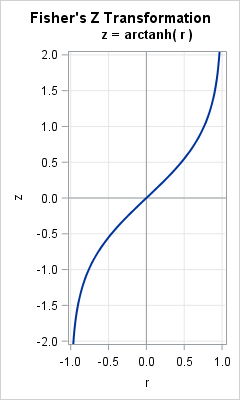Pearson's correlation measures the linear association between two variables. Because the correlation is bounded between [-1, 1], the sampling distribution for highly correlated variables is highly skewed. Even for bivariate normal data, the skewness makes it challenging to estimate confidence intervals for the correlation, to run one-sample hypothesis tests ("Is the correlation equal to 0.5?"), and to run two-sample hypothesis tests ("Do these two samples have the same correlation?").

In 1921, R. A. Fisher studied the correlation of bivariate normal data and discovered a wonderful transformation (shown to the right) that converts the skewed distribution of the sample correlation (r) into a distribution that is approximately normal. Furthermore, whereas the variance of the sampling distribution of r depends on the correlation, the variance of the transformed distribution is independent of the correlation. The transformation is called Fisher's z transformation. This article describes Fisher's z transformation and shows how it transforms a skewed distribution into a normal distribution.

### The distribution of the sample correlation

The following graph (click to enlarge) shows the sampling distribution of the correlation coefficient for bivariate normal samples of size 20 for four values of the population correlation, rho (ρ). You can see that the distributions are very skewed when the correlation is large in magnitude.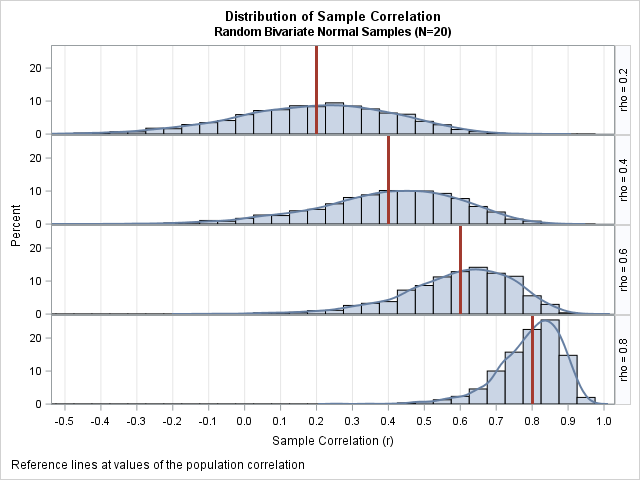The graph was created by using simulated bivariate normal data as follows:

1. For rho=0.2, generate M random samples of size 20 from a bivariate normal distribution with correlation rho. (For this graph, M=2500.)
2. For each sample, compute the Pearson correlation.
3. Plot a histogram of the M correlations.
4. Overlay a kernel density estimate on the histogram and add a reference line to indicate the correlation in the population.
5. Repeat the process for rho=0.4, 0.6, and 0.8.

The histograms approximate the sampling distribution of the correlation coefficient (for bivariate normal samples of size 20) for the various values of the population correlation. The distributions are not simple. Notice that the variance and the skewness of the distributions depend on the value the underlying correlation (ρ) in the population.

### Fisher's transformation of the correlation coefficient

Fisher sought to transform these distributions into normal distributions. He proposed the transformation f(r) = arctanh(r), which is the inverse hyperbolic tangent function. The graph of arctanh is shown at the top of this article. Fisher's transformation can also be written as (1/2)log( (1+r)/(1-r) ). This transformation is sometimes called Fisher's "z transformation" because the letter z is used to represent the transformed correlation: z = arctanh(r).

How he came up with that transformation is a mystery to me, but he was able to show that arctanh is a normalizing and variance-stabilizing transformation. That is, when r is the sample correlation for bivariate normal data and z = arctanh(r) then the following statements are true (See Fisher, Statistical Methods for Research Workers, 6th Ed, pp 199-203):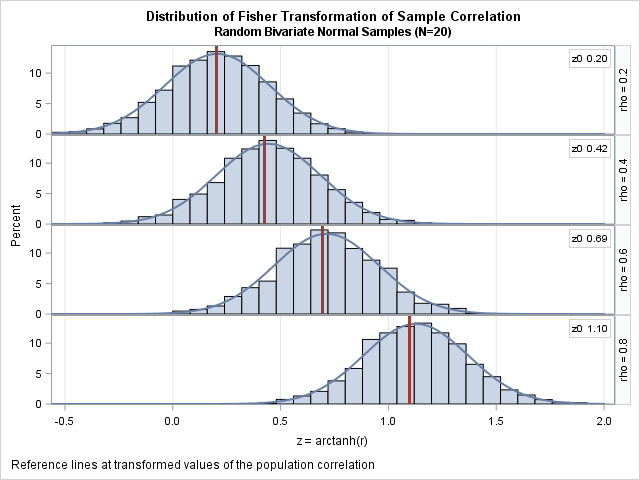• The distribution of z is approximately normal and "tends to normality rapidly as the sample is increased" (p 201).
• The standard error of z is approximately 1/sqrt(N-3), which is independent of the value of the correlation.

The graph to the right demonstrates these statements. The graph is similar to the preceding panel, except these histograms show the distributions of the transformed correlations z = arctanh(r). In each cell, the vertical line is drawn at the value arctanh(ρ). The curves are normal density estimates with σ = 1/sqrt(N-3), where N=20.

The two features of the transformed variables are apparent. First, the distributions are normally distributed, or, to quote Fisher, "come so close to it, even for a small sample..., that the eye cannot detect the difference" (p. 202). Second, the variance of these distributions are constant and are independent of the underlying correlation.

### Fisher's transformation and confidence intervals

From the graph of the transformed variables, it is clear why Fisher's transformation is important. If you want to test some hypothesis about the correlation, the test can be conducted in the z coordinates where all distributions are normal with a known variance. Similarly, if you want to compute a confidence interval, the computation can be made in the z coordinates and the results "back transformed" by using the inverse transformation, which is r = tanh(z).

You can perform the calculations by applying the standard formulas for normal distributions (see p. 3-4 of Shen and Lu (2006)), but most statistical software provides an option to use the Fisher transformation to compute confidence intervals and to test hypotheses. In SAS, the CORR procedure supports the FISHER option to compute confidence intervals and to test hypotheses for the correlation coefficient.

The following call to PROC CORR computes a sample correlation between the length and width of petals for 50 Iris versicolor flowers. The FISHER option specifies that the output should include confidence intervals based on Fisher's transformation. The RHO0= suboption tests the null hypothesis that the correlation in the population is 0.75. (The BIASADJ= suboption turns off a bias adjustment; a discussion of the bias in the Pearson estimate will have to wait for another article.)

```proc corr data=sashelp.iris fisher(rho0=0.75 biasadj=no); where Species='Versicolor'; var PetalLength PetalWidth; run;```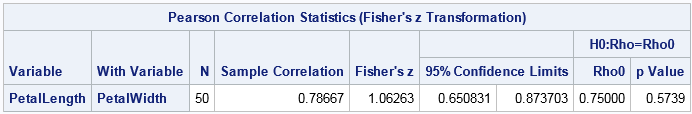The output shows that the Pearson estimate is r=0.787. A 95% confidence interval for the correlation is [0.651, 0.874]. Notice that r is not the midpoint of that interval. In the transformed coordinates, z = arctanh(0.787) = 1.06 is the center of a symmetric confidence interval (based on a normal distribution with standard error 1/sqrt(N-3)). However, the inverse transformation (tanh) is nonlinear, and the right half-interval gets compressed more than the left half-interval.

For the hypothesis test of ρ = 0.75, the output shows that the p-value is 0.574. The data do not provide evidence to reject the hypothesis that ρ = 0.75 at the 0.05 significance level. The computations for the hypothesis test use only the transformed (z) coordinates.

### Summary

This article shows that Fisher's "z transformation," which is z = arctanh(r), is a normalizing transformation for the Pearson correlation of bivariate normal samples of size N. The transformation converts the skewed and bounded sampling distribution of r into a normal distribution for z. The standard error of the transformed distribution is 1/sqrt(N-3), which does not depend on the correlation. You can perform hypothesis tests in the z coordinates. You can also form confidence intervals in the z coordinates and use the inverse transformation (r=tanh(z)) to obtain a confidence interval for ρ.

The Fisher transformation is exceptionally useful for small sample sizes because, as shown in this article, the sampling distribution of the Pearson correlation is highly skewed for small N. When N is large, the sampling distribution of the Pearson correlation is approximately normal except for extreme correlations. Although the theory behind the Fisher transformation assumes that the data are bivariate normal, in practice the Fisher transformation is useful as long as the data are not too skewed and do not contain extreme outliers.

The post Fisher's transformation of the correlation coefficient appeared first on The DO Loop.If you use SAS regression procedures, you are probably familiar with the "stars and bars" notation, which enables you to construct interaction effects in regression models. Although you can construct many regression models by using that classical notation, a friend recently reminded me that the EFFECT statement in SAS provides greater control over the interaction terms in a regression model.

The EFFECT statement is supported in many SAS procedures, including the GLIMMIX, GLMSELECT, and LOGISTIC procedures. Because those procedures can output a design matrix, you can use a model that is generated by an EFFECT statement in any SAS procedure, even older procedures that do not support the EFFECT statement. I have previously shown how you can use the SPLINE option in the EFFECT statement to generate spline effects. This article deals with polynomial effects, which are effects that are formed by elementwise multiplication of continuous variables, such as x1*x1, x1*x2, and x1*x1*x2*x3.

The following statements rename a few continuous variables in the Sashelp.Heart data set, which will be used in the examples. The new variables (x1, x2, x3, and x4) are easier to type and using these short variable names makes it easier to see how interactions effects are formed.

```data Heart / view=Heart; set Sashelp.Heart; rename Height=x1 Weight=x2 Diastolic=x3 Systolic=x4; run;```

### A review of "stars and bars" notation in SAS

Recall that many SAS regression procedures (such as GLM, GENMOD, LOGISTIC, MIXED,...) support the bar operator (|) to specify interactions between effects. For example, the following MODEL statement specifies that the model should include all main effects and all higher-order interactions:

```proc logistic data=Heart; model Y = x1 | x2 | x3 | x4; /* all main effects and interactions up to 4-way */ run;```

The previous MODEL statement includes all two-way, three-way, and four-way interaction effects between distinct variables. In practice, fitting a model with so many effects will lead to overfitting, so most analysts restrict the model to two-way interactions. In SAS you can use the "at" operator (@) to specify the highest interaction terms in the model. For example, the following syntax specifies that the model contains only main effects and two-way interactions:

```model Y = x1 | x2 | x3 | x4 @2; /* main effects and two-way interactions */ /* equivalent: model Y = x1 x2 x3 x4 x1*x2 x1*x3 x1*x4 x2*x3 x2*x4 x3*x4; */```

### Use the EFFECT statement to build polynomial effects

Notice that the bar operator does not generate the interaction of a variable with itself. For example, the terms x1*x1 and x2*x2 are not generated. Notice also that you need to explicitly type out each variable when you use the bar operator. You cannot use "colon notation" (x:) or hyphens (x1-x4) to specify a range of variables. For four variable, this is an inconvenience; for hundreds of variables, this is a serious problem.

The EFFECT statement enables you to create polynomial effects, which have the following advantages:

• You can use colon notation or a hyphen to specify a range of variables. (You can also specify a space-separated list of variable names.)
• You can control the degree of the polynomial terms and the maximum value of the exponent that appears in each term.
• You can generate interactions between a variable and itself.

The POLYNOMIAL option in the EFFECT statement is described in terms of multivariate polynomials. Recall that a multivariate monomial is a product of powers of variables that have nonnegative integer exponents. For example, x2 y z is a monomial that contains three variables. The degree of a monomial is the sum of the exponents of the variables. The previous monomial has degree 4 because 4 = 2 + 1 + 1.

### Use the EFFECT statement to generate two-way interactions

The syntax for the EFFECT statement is simple. For example, to generate all main effects and two-way interactions, including second-degree terms like x1*x1 and x2*x2, use the following syntax:

```ods select ParameterEstimates(persist); proc logistic data=Heart; effect poly2 = polynomial(x1-x4 / degree=2); model Status = poly2; /* equivalent: model Status = x1 | x2 | x3 | x4 @2 x1*x1 x2*x2 x3*x3 x4*x4; */ run;```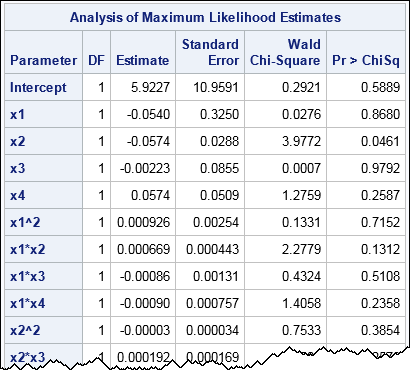The name of the effect is 'poly2'. It is a polynomial effect that contains all terms that involve first- and second-degree monomials. Thus it contains the main effects, the two-way interactions between variables, and the terms x1*x1, x2*x2, x3*x3, and x4*x4. The equivalent model in "stars and bars" notation is shown in the comment. The models are the same, although the variables are listed in a different order.

You can also use the colon operator to select variables that have a common prefix. For example, to specify all polynomial effects for variables that begin with the prefix 'x', you can use EFFECT poly2 = POLYNOMIAL(x: / DEGREE=2).

You can use the MDEGREE= option to control the maximum degree of any exponent in a monomial. For example, the monomials x1*x1 and x1*x2 are both second-degree monomials, but the maximum exponent that appears in the first monomial is 2 whereas the maximum exponent that appears in the second monomial is 1. The following syntax generates only monomial terms for which the maximum exponent is 1, which means that x1*x1 and x2*x2 will not appear:

```proc logistic data=Heart; effect poly21 = polynomial(x1-x4 / degree=2 mdegree=1); /* exclude x1*x1, x2*x2, etc. */ model Status = poly21; /* equivalent: model Status = x1 | x2 | x3 | x4 @2 */ run;```

### Use the EFFECT statement to generate two-way interactions between lists of variables

A novel use of polynomial effects is to generate all two-way interactions between variables in one list and variables in another list. For example, suppose you are interested in the interactions between the lists (x1 x2) and (x3 x4), but you are not interested in within-list interactions such as x1*x2 and x3*x4. By using polynomial effects, you can create the two lists and then use the bar operator to request all main and two-way interactions between elements of the lists, as follows:

```proc logistic data=Heart; effect list1 = polynomial(x1-x2); /* first list of variables */ effect list2 = polynomial(x3-x4); /* second list of variables */ model Status = list1 | list2; /* main effects and pairwise interactions between lists */ /* equivalent: model Status = x1 x2 x3 x4 x1*x3 x1*x4 x2*x3 x2*x4; */ run;```

Notice that you can use the EFFECT statement multiple times in the same procedure. This is a powerful technique! By using multiple EFFECT statements, you can name sets of variables that have similar properties, such as demographic effects (age, sex), lifestyle effects (diet, exercise), and physiological effects (blood pressure, cholesterol). You can then easily construct models that contain interactions between these sets, such as LIFESTYLE | PHYSIOLOGY.

In summary, the POLYNOMIAL option in the EFFECT statement enables you to control the interactions between variables in a model. You can form models that are equivalent to the "stars and bars" syntax or specify more complex models. In particular, you can use the EFFECT statement multiple times to construct lists of variables and interactions between elements of the lists. For more information about polynomial effects, see the SAS documentation for the POLYNOMIAL option in the EFFECT STATEMENT.

The post Construct polynomial effects in SAS regression models appeared first on The DO Loop.Correlation is a fundamental statistical concept that measures the linear association between two variables. There are multiple ways to think about correlation: geometrically, algebraically, with matrices, with vectors, with regression, and more. To paraphrase the great songwriter Paul Simon, there must be 50 ways to view your correlation! But don't "slip out the back, Jack," this article describes only seven of them.

How can we understand these many different interpretations? As the song says, "the answer is easy if you take it logically." These seven ways to view your Pearson correlation are based on the wonderful paper by Rodgers and Nicewander (1988), "Thirteen ways to look at the correlation coefficient," which I recommend for further reading.

### 1. GraphicallyThe simplest way to visualize correlation is to create a scatter plot of the two variables. A typical example is shown to the right. (Click to enlarge.) The graph shows the heights and weights of 19 students. The variables have a strong linear "co-relation," which is Galton's original spelling for "correlation." For these data, the Pearson correlation is r = 0.8778, although few people can guess that value by looking only at the graph.

For data that are approximately bivariate normal, the points will align northwest-to-southeast when the correlation is negative and will align southwest-to-northeast when the data are positively correlated. If the point-cloud is an amorphous blob, the correlation is close to zero.

In reality, most data are not well-approximated by a bivariate normal distribution. Furthermore, Anscombe's Quartet provides a famous example of four point-clouds that have exactly the same correlation but very different appearances. (See also the images in the Wikipedia article about correlation.) So although a graphical visualization can give you a rough estimate of a correlation, you need computations for an accurate estimate.

### 2. The sum of crossproducts

In elementary statistics classes, the Pearson sample correlation coefficient between two variables x and y is usually given as a formula that involves sums. The numerator of the formula is the sum of crossproducts of the centered variables. The denominator involves the sums of squared deviations for each variable. In symbols: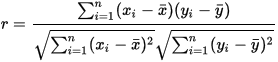The terms in the numerator involve the first central moments and the terms in the denominator involve the second central moments. Consequently, Pearson's correlation is sometimes called the product-moment correlation.

### 3. The inner product of standardized vectors

I have a hard time remembering complicated algebraic formulas. Instead, I try to visualize problems geometrically. The way I remember the correlation formula is as the inner (dot) product between two centered and standardized vectors. In vector notation, the centered vectors are x - x̄ and y - ȳ. A vector is standardized by dividing by its length, which in the Euclidean norm is the square root of the sum of the square of the elements. Therefore you can define u = (x - x̄) / || x - x̄ || and v = (y - ȳ) / || y - ȳ ||. Looking back at the equation in the previous section, you can see that the correlation between the vectors x and y is the inner product r = u · v. This formula shows that the correlation coefficient is inavariant under affine transformations of the data.

### 4. The angle between two vectors

Linear algebra teaches that the inner product of two vectors is related to the angle between the vectors. Specifically, u · v = ||u|| ||v|| cos(θ), where θ is the angle between the vectors u and v. Dividing both sides by the lengths of u and v and using the equations in the previous section, we find that r = cos(θ), where θ is the angle between the vectors.

This equation gives two important facts about the Pearson correlation. First, the correlation is bounded in the interval [-1, 1]. Second, it gives the correlation for three important geometric cases. When x and y have the same direction (θ = 0), then their correlation is +1. When x and y have opposite directions (θ = π), then their correlation is -1. When x and y are orthogonal (θ = π/2), their correlation is 0.

### 5. The standardized covariance

Recall that the covariance between two variables x and y is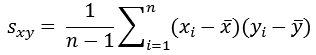The covariance between two variables depends on the scales of measurement, so the covariance is not a useful statistic for comparing the linear association. However, if you divide by the standard deviation of each variable, then the variables become dimensionless. Recall that the standard deviation is the square root of the variance, which for the x variable is given by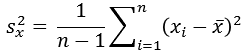Consequently, the expression sxy / (sx sy) is a standardized covariance. If you expand the terms algebraically, the "n - 1" terms cancel and you are left with the Pearson correlation.

### 6. The slope of the regression line between two standardized variables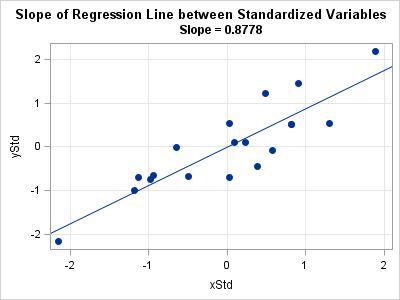Most textbooks for elementary statistics mention that the correlation coefficient is related to the slope of the regression line that estimates y from x. If b is the slope, then the result is r = b (sx / sy). That's a messy formula, but if you standardize the x and y variables, then the standard deviations are unity and the correlation equals the slope.

This fact is demonstrated by using the same height and weight data for 19 students. The graph to the right shows the data for the standardized variables, along with an overlay of the least squares regression line. The slope of the regression line is 0.8778, which is the same as the correlation between the variables.

### 7. Geometric mean of regression slopes

The previous section showed a relationship between two of the most important concepts in statistics: correlation and regression. Interestingly, the correlation is also related to another fundamental concept, the mean. Specifically, when two variables are positively correlated, the correlation coefficient is equal to the geometric mean of two regression slopes: the slope of y regressed on x (bx) and the slope of x regressed on y (by).

To derive this result, start from the equation in the previous section, which is r = bx (sx / sy). By symmetry, it is also true that r = by (sy / sx). Consequently, r2 = bx by or r = sqrt( bx by ), which is the geometric mean of the two slopes..

### Summary

This article shows multiple ways that you can view a correlation coefficient: analytically, geometrically, via linear algebra, and more. The Rodgers and Nicewander (1988) article includes other ideas, some of which are valid only asymptotically or approximately.

If you want to see the computations for these methods applied to real data, you can download a SAS program that produces the computations and graphs in this article.

The post 7 ways to view correlation appeared first on The DO Loop.Aa previous article discussed the mathematical properties of the singular value decomposition (SVD) and showed how to use the SVD subroutine in SAS/IML software. This article uses the SVD to construct a low-rank approximation to an image. Applications include image compression and denoising an image.

### Construct a grayscale image

The value of each pixel in a grayscale image can be stored in a matrix where each element of the matrix is a value between 0 (off) and 1 (full intensity). I want to create a small example that is easy to view, so I'll create a small matrix that contains information for a low-resolution image of the capital letters "SVD." Each letter will be five pixels high and three pixels wide, arranges in a 7 x 13 array of 0/1 values. The following SAS/IML program creates the array and displays a heat map of the data:

```ods graphics / width=390px height=210px; proc iml; SVD = {0 0 0 0 0 0 0 0 0 0 0 0 0, 0 1 1 1 0 1 0 1 0 1 1 0 0, 0 1 0 0 0 1 0 1 0 1 0 1 0, 0 1 1 1 0 1 0 1 0 1 0 1 0, 0 0 0 1 0 1 0 1 0 1 0 1 0, 0 1 1 1 0 0 1 0 0 1 1 0 0, 0 0 0 0 0 0 0 0 0 0 0 0 0 }; A = SVD; /* ColorRamp: White Gray1 Gray2 Gray3 Black */ ramp = {CXFFFFFF CXF0F0F0 CXBDBDBD CX636363 CX000000}; call heatmapcont(A) colorramp=ramp showlegend=0 title="Original Image" range={0,1};```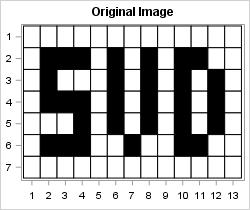### A low-rank approximation to an image

Because the data matrix contains only five non-zero rows, the rank of the A matrix cannot be more than 5. The following statements compute the SVD of the data matrix and create a plot of the singular values. The plot of the singular values is similar to the scree plot in principal component analysis, and you can use the plot to help choose the number of components to retain when approximating the data.

```call svd(U, D, V, A); /* A = U*diag(D)*V` */ title "Singular Values"; call series(1:nrow(D), D) grid={x y} xvalues=1:nrow(D) label={"Component" "Singular Value"};```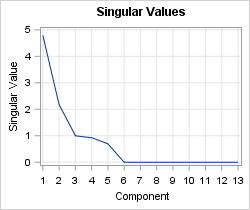For this example, it looks like retaining three or five components would be good choices for approximating the data. To see how low-rank approximations work, let's generate and view the rank-1, rank-2, and rank-3 approximations:

```keep = 1:3; /* use keep=1:5 to see all approximations */ do i = 1 to ncol(keep); idx = 1:keep[i]; /* components to keep */ Ak = U[ ,idx] * diag(D[idx]) * V[ ,idx]`; /* rank k approximation */ Ak = (Ak - min(Ak)) / range(Ak); /* for plotting, stdize into [0,1] */ s = "Rank = " + char(keep[i],1); call heatmapcont(Ak) colorramp=ramp showlegend=0 title=s range={0, 1}; end;```The rank-1 approximation does a good job of determining the columns and do not contain that contain the letters. The approximation also picks out two rows that contain the horizontal strokes of the capital "S."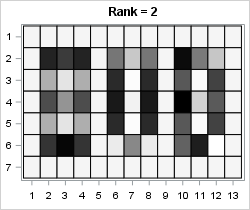The rank-2 approximation refines the image and adds additional details. You can begin to make out the letters "SVD." In particular, all three horizontal strokes for the "S" are visible and you can begin to see the hole in the capital "D."The rank-3 approximation contains enough details that someone unfamiliar with the message can read it. The "S" is reconstructed almost perfectly and the space inside the "V" and "D" is almost empty. Even though this data is five-dimensional, this three-dimensional approximation is very good.

Not only is a low-rank approximation easier to work with than the original five-dimensional data, but a low-rank approximation represents a compression of the data. The original image contains 7 x 13 = 91 values. For the rank-3 approximation, three columns of the U matrix contain 33 numbers and three columns of VT contain 15 numbers. So the total number of values required to represent the rank-3 approximation is only 48, which is almost half the number of values as for the original image.

### Denoising an image

You can use the singular value decomposition and low-rank approximations to try to eliminate random noise that has corrupted an image. Every TV detective series has shown an episode in which the police obtain a blurry image of a suspect's face or license plate. The detective asks the computer technician if she can enhance the image. With the push of a button, the blurry image is replaced by a crystal clear image that enables the police to identify and apprehend the criminal.

The image reconstruction algorithms used in modern law enforcement are more sophisticated than the SVD. Nevertheless, the SVD can do a reasonable job of removing small random noise from an image, thereby making certain features easier to see. The SVD has to have enough data to work with, so the following statements duplicate the "SVD" image four times before adding random Gaussian noise to the data. The noise has a standard deviation equal to 25% of the range of the data. The noisy data is displayed as a heat map on the range [-0.25, 1.25] by using a color ramp that includes yellow for negative values and blue for values greater than 1.

```call randseed(12345); A = (SVD // SVD) || (SVD // SVD); /* duplicate the image */ A = A + randfun( dimension(A), "Normal", 0, 0.25); /* add Gaussian noise */ /* Yellow White Gray1 Gray2 Gray3 Black Blue */ ramp2 = {CXFFEDA0 CXFFFFFF CXF0F0F0 CXBDBDBD CX636363 CX000000 CX3182BD}; call heatmapcont(A) colorramp=ramp2 showlegend=0 title="Noisy Image" range={-0.5, 1.5};```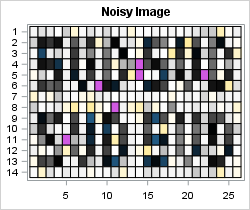I think most police officers would be able to read this message in spite of the added noise, but let's see if the SVD can clean it up. The following statements compute the SVD and create a plot of the singular values:

```call svd(U, D, V, A); /* A = U*diag(D)*V` */ call series(1:nrow(D), D) grid={x y} xvalues=1:nrow(D) label={"Component" "Singular Value"};```There are 14 non-zero singular values. In theory, the main signal is contained in the components that correspond to the largest singular values whereas the noise is captured by the components for the smallest singular values. For these data, the plot of the singular values suggests that three or five (or nine) components might capture the main signal while ignoring the noise. The following statements create and display the rank-3 and rank-5 approximations. Only the rank-5 approximation is shown.

```keep = {3 5}; /* which components to examine? */ do i = 1 to ncol(keep); idx = 1:keep[i]; Ak = U[ ,idx] * diag(D[idx]) * V[ ,idx]`; Ak = (Ak - min(Ak)) / range(Ak); /* for plotting, standardize into [0,1] */ s = "Rank = " + char(keep[i],2); call heatmapcont(Ak) colorramp=ramp showlegend=0 title=s range={0, 1}; end;```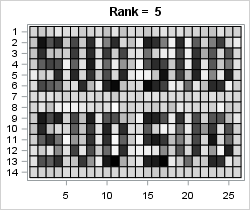The denoised low-rank image is not as clear as Hollywood depicts, but it is quite readable. It is certainly good enough to identify a potential suspect. I can hear the detective mutter, "Book 'em, Danno! Murder One."

### Summary and further reading

In summary, the singular value decomposition (SVD) enables you to approximate a data matrix by using a low-rank approximation. This article uses a small example for which the full data matrix is rank-5. A plot of the singular values can help you choose the number of components to retain. For this example, a rank-3 approximation represents the essential features of the data matrix.

For similar analyses and examples that use the singular value decomposition, see

In SAS software, the SVD is heavily used in the SAS Text Miner product. For an overview of how a company can use text mining to analyze customer support issues, see Sanders and DeVault (2004) "Using SAS at SAS: The Mining of SAS Technical Support."

The post The singular value decomposition and low-rank approximations appeared first on The DO Loop.Visualizing the correlations between variables often provides insight into the relationships between variables. I've previously written about how to use a heat map to visualize a correlation matrix in SAS/IML, and Chris Hemedinger showed how to use Base SAS to visualize correlations between variables.

Recently a SAS programmer asked how to construct a bar chart that displays the pairwise correlations between variables. This visualization enables you to quickly identify pairs of variables that have large negative correlations, large positive correlations, and insignificant correlations.

In SAS, PROC CORR can computes the correlations between variables, which are stored in matrix form in the output data set. The following call to PROC CORR analyzes the correlations between all pairs of numeric variables in the Sashelp.Heart data set, which contains data for 5,209 patients in a medical study of heart disease. Because of missing values, some pairwise correlations use more observations than others.

```ods exclude all; proc corr data=sashelp.Heart; /* pairwise correlation */ var _NUMERIC_; ods output PearsonCorr = Corr; /* write correlations, p-values, and sample sizes to data set */ run; ods exclude none;```

The CORR data set contains the correlation matrix, p-values, and samples sizes. The statistics are stored in "wide form," with few rows and many columns. As I previously discussed, you can use the HEATMAPCONT subroutine in SAS/IML to quickly visualize the correlation matrix:

```proc iml; use Corr; read all var "Variable" into ColNames; /* get names of variables */ read all var (ColNames) into mCorr; /* matrix of correlations */ ProbNames = "P"+ColNames; /* variables for p-values are named PX, PY, PZ, etc */ read all var (ProbNames) into mProb; /* matrix of p-values */ close Corr;   call HeatmapCont(mCorr) xvalues=ColNames yvalues=ColNames colorramp="ThreeColor" range={-1 1} title="Pairwise Correlation Matrix";```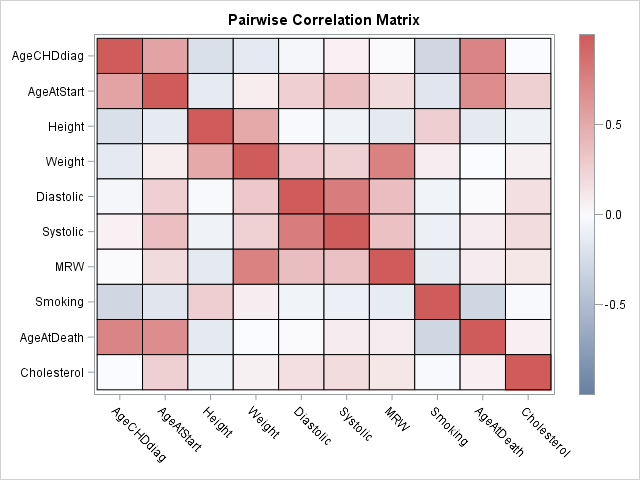The heat map gives an overall impression of the correlations between variables, but it has some shortcomings. First, you can't determine the magnitudes of the correlations with much precision. Second, it is difficult to compare the relative sizes of correlations. For example, which is stronger: the correlation between systolic and diastolic blood pressure or the correlation between weight and MRW? (MRW is a body-weight index.)

These shortcomings are resolved if you present the pairwise correlations as a bar chart. To create a bar chart, it is necessary to convert the output into "long form." Each row in the new data set will represent a pairwise correlation. To identify the row, you should also create a new variable that identifies the two variables whose correlation is represented. Because the correlation matrix is symmetric and has 1 on the diagonal, the long-form data set only needs the statistics for the lower-triangular portion of the correlation matrix.

Let's extract the data in SAS/IML. The following statements construct a new ID variable that identifies each new row and extract the correlations and p-values for the lower-triangular elements. The statistics are written to a SAS data set called CorrPairs. (In Base SAS, you can transform the lower-triangular statistics by using the DATA step and arrays, similar to the approach in this SAS note; feel free to post your Base SAS code in the comments.)

```numCols = ncol(mCorr); /* number of variables */ numPairs = numCols*(numCols-1) / 2; length = 2*nleng(ColNames) + 5; /* max length of new ID variable */ PairNames = j(NumPairs, 1, BlankStr(length)); i = 1; do row= 2 to numCols; /* construct the pairwise names */ do col = 1 to row-1; PairNames[i] = strip(ColNames[col]) + " vs. " + strip(ColNames[row]); i = i + 1; end; end;   lowerIdx = loc(row(mCorr) > col(mCorr)); /* indices of lower-triangular elements */ Corr = mCorr[ lowerIdx ]; Prob = mProb[ lowerIdx ]; Significant = choose(Prob > 0.05, "No ", "Yes"); /* use alpha=0.05 signif level */   create CorrPairs var {"PairNames" "Corr" "Prob" "Significant"}; append; close; QUIT;```

You can use the HBAR statement in PROC SGPLOT to construct the bar chart. This bar chart contains 45 rows, so you need to make the graph tall and use a small font to fit all the labels without overlapping. The call to PROC SORT and the DISCRETEORDER=DATA option on the YAXIS statement ensure that the categories are displayed in order of increasing correlation.

```proc sort data=CorrPairs; by Corr; run;   ods graphics / width=600px height=800px; title "Pairwise Correlations"; proc sgplot data=CorrPairs; hbar PairNames / response=Corr group=Significant; refline 0 / axis=x; yaxis discreteorder=data display=(nolabel) labelattrs=(size=6pt) fitpolicy=none offsetmin=0.012 offsetmax=0.012 /* half of 1/k, where k=number of catgories */ colorbands=even colorbandsattrs=(color=gray transparency=0.9); xaxis grid display=(nolabel); keylegend / position=topright location=inside across=1; run;```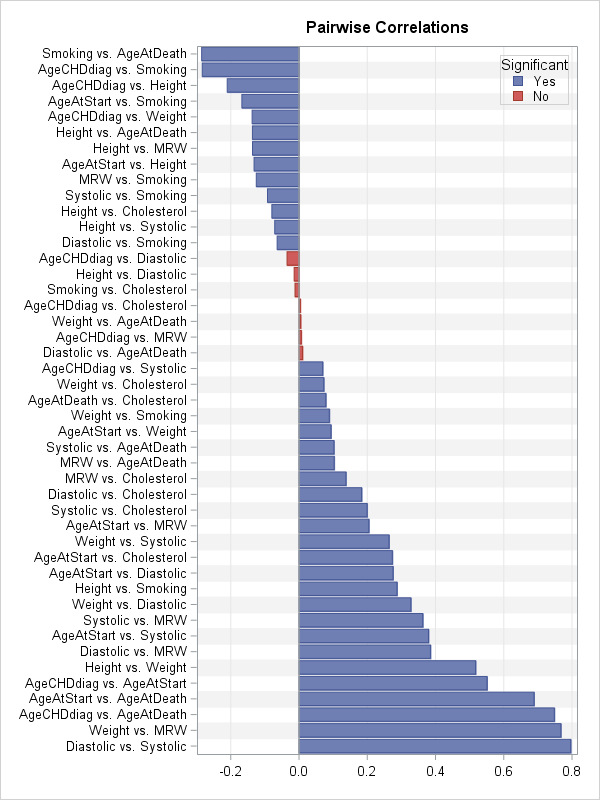The bar chart (click to enlarge) enables you to see which pairs of variables are highly correlated (positively and negatively) and which have correlations that are not significantly different from 0. You can use additional colors or reference lines if you want to visually emphasize other features, such as the correlations that are larger than 0.25 in absolute value.

The bar chart is not perfect. This example, which analyzes 10 variables, is very tall with 45 rows. Among k variables there are k(k-1)/2 correlations, so the number of pairwise correlations (rows) increases quadratically with the number of variables. In practice, this chart would be unreasonably tall when there are 14 or 15 variables (about 100 rows).

Nevertheless, for 10 or fewer variables, a bar chart of the pairwise correlations provides an alternative visualization that has some advantages over a heat map of the correlation matrix. What do you think? Would this graph be useful in your work? Leave a comment.

The post Use a bar chart to visualize pairwise correlations appeared first on The DO Loop.